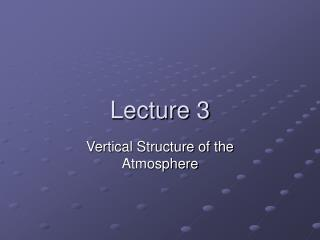DownloadDownload PresentationLecture 3

# Lecture 3

Télécharger la présentation## Lecture 3

- - - - - - - - - - - - - - - - - - - - - - - - - - - E N D - - - - - - - - - - - - - - - - - - - - - - - - - - -
##### Presentation Transcript

1. Lecture 3 Vertical Structure of the Atmosphere

2. Average Vertical Temperature profile

3. Atmospheric Layers • Troposphere • On average, temperature decreases with height • Stratosphere • On average, temperature increases with height • Mesosphere • Thermosphere

4. Lapse Rate • Lapse rate is rate that temperature decreases with height

5. Soundings • Actual vertical temperature profiles are called soundings • A sounding is obtained using an instrument package called a radiosonde • Radiosondes are carried aloft using balloons filled with hydrogen or helium

6. Application: Reduction to Sea Level(See Ahrens, Ch. 6) proportional to weight of this column of air Surface pressure also called station pressure (if there is a weather station there!) Surface pressure here

7. Math Obtained by integrating the hydrostatic equation from the surface to top of atmosphere.

8. Deficiencies of Surface Pressure • Spatial variations in surface pressure mainly due to topography, not meteorology

9. Height contours on topographic map Units: m It’s a mountain! 1050 1000 950 900

10. Put a bunch of barometers on the mountain.

11. Surface pressure (approximately) Units: hPa 885 890 895 900 Isobar pattern looks just like height-contour pattern!

12. “Reduction to Sea Level” is proportional to weight of this column of air Let T = sfc. temp. (12-hour avg.) Surface pressure here For sea level pressure add weight of isothermal column of air temp = T. Sea Level

13. Pressure as Vertical Coordinate • Pressure is a 1-1 function of height • i.e., a given pressure occurs at a unique height • Thus, the pressure can be used to specify the vertical position of a point

14. Pressure Surfaces • Let the pressure, p1, be given. • At a given instant, consider all points (x, y, z) where p = p1 • This set of points defines a surface

15. z p = p1 z(x2) z(x1) x x1 x2

16. Height Contours Heights indicated in dekameters (dam) 1dam = 10m

17. Two Pressure Surfaces z p = p2 z2 z2 – z1 z1 p = p1

18. Thickness • z2 – z1 is called the thickness of the layer • Hypsometric equation  thickness proportional to mean temperature of layer

19. Thickness Gradients z p = p2 Large thickness Small thickness warm Cold p = p1

20. Exercise • Suppose that the mean temperature between 1000 hPa and 500 hPa is -10C. • Calculate the thickness (in dam)

21. Repeat, for T = -20C

22. Thickness Maps